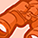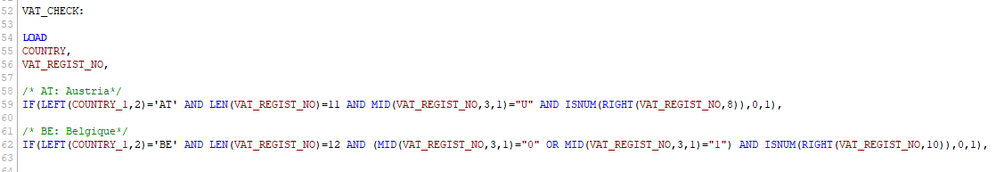# QlikView App Dev

Discussion Board for collaboration related to QlikView App Development.

Announcements
Customer & Partners, DEC. 9, 11 AM ET: Qlik Product & Strategy Roadmap Session: Data Analytics REGISTER NOW
cancel
Showing results for
Did you mean:Creator II

## Multiple If statement in Script

Hello all,

I  wrote many If statement in a script . Then, I would like to sum the value of  all my "else" statements.

But I don't know how I can recuperate in an unique variable the sum total of the values of my "else" statement.

Please could you help me to find a way to make the sum of all the "else" statement.

Below, the beginning of my script:Labels (2)

• ### QlikView scripting

1 Solution

Accepted SolutionsCreator

I thought I had replied to this one, now I'm wondering where I posted the reply, the other person is probably now very confused about my response which bears no relation to their question 🙂

Anyway, I'd do this in a single statement first, but then split it out in a preceding load to make the expressions easier:

,IF(VAT_CHECK='OK',1) AS VATOK

,IF(VAT_CHECK='NO',1) AS VATNOTOK;

,VAT

,IF(CTRY='AT' AND LEN(VAT)=11 AND ISNUM(RIGHT(VAT,8))

OR CTRY='BE' AND LEN(VAT)=12 AND ISNUM(RIGHT(VAT,5))

OR CTRY='UK' AND LEN(VAT)=10 AND ISNUM(RIGHT(VAT,4))

, 'OK'

, 'NO' ) AS VAT_CHECK

FROM.......

Then you can use an expression such as SUM(VATOK) and/or SUM(VATNOTOK).

Clear??

8 RepliesCreator

The script seems odd in that either you have the IF statement wrong or you are missing the AS part of the statement.

What I mean is:

IF(LEFT(CTRY,2)='AT' AND LEN(VAT)=11 AND MID(VAT,3,1)="U" AND ISNUM(RIGHT(VAT,8)),0,1) AS AUSTRIA_VALUE

IF(LEFT(CTRY,2)='BE' AND LEN(VAT)=11 AND MID(VAT,3,1)="U" AND ISNUM(RIGHT(VAT,8)),0,1) AS BELGIUM_VALUE

Or you should have one nested IF...THEN...ELSE statement such as:

IF(LEFT(CTRY,2)='AT' AND LEN(VAT)=11 AND MID(VAT,3,1)="U" AND ISNUM(RIGHT(VAT,8)),0,

IF(LEFT(CTRY,2)='BE' AND LEN(VAT)=11 AND MID(VAT,3,1)="U" AND ISNUM(RIGHT(VAT,8)),0,1)) AS CTRY_VAT_VALUE

Now, if you are really trying to do the first example then you can do a preceding load on that load as follows:

, IF(AUSTRIA_VALUE=0 AND BELGIUM_VALUE=0,1,0) AS ALL_ZEROS;

VAT,

IF(LEFT(CTRY,2)='AT' AND LEN(VAT)=11 AND MID(VAT,3,1)="U" AND ISNUM(RIGHT(VAT,8)),0,1) AS AUSTRIA_VALUE,

IF(LEFT(CTRY,2)='BE' AND LEN(VAT)=11 AND MID(VAT,3,1)="U" AND ISNUM(RIGHT(VAT,8)),0,1) AS BELGIUM_VALUE

FROM........

If you are trying to do the nested if....then....else then of course your final value in the statement is the final else.

IF(a=1 and b=2 and c=3,0,

if(a=2 and b=3 and c=4,0,

if(g=6 and f=4 and h=9,0,

1))) AS Some_ValueCreator II
Author

Hello @NW1965 ,

If I understand well what you said in your reply: It's necessary to name each if statement in a first table.

So, in this way, I could in another table call the if statement and realize the sum.

My purpose is to count for each country the sum where value equal to 1 in the if statement.In the first table, I realized all my if statements.

Then, in a second table, I tried to count the if equal to "1" but when I run my script the load of this table failed.

Please could you help me to count the if equal to "1"  in the second table thanks to the variables in the 1st table.

I hope that my explanation are clear...Creator
OK, that won't work because you can't do a sum without grouping in a load statement, you should remember that you are loading one row at a time. Perhaps what you need to do is explain what you want to achieve rather than trying to explain part of the solution and part of the requirement. Questions: 1. In the VAT_REGEX table you are going to create rows of data where you will have a field called AT_VAT and another field called BE_VAT, those fields will contain a 0 or a 1, what is the business purpose or meaning of those fields 2. In the VAT_CHECK table you are creating one more field, this one called TOTAL (I'd recommend renaming that as TOTAL is also a keyword in QlikView), bearing in mind that this is a transactional table with only one country defined per row, this field will only ever hold a value of 1 or 0, so I'd be interested to know the purpose of this too 3. If this really is what you want then the TOTAL field should actually just be defined as IF(AT_VAT=1 OR BE_VAT=1,1) AS TOTAL (i.e. remove the SUM from this) 4. Also be aware the if you already have CUSTOMER_TEST as a table then you have VAT_REGEX and then VAT_CHECK and all of these tables have COUNTRY and VAT_REGIST fields, you're going to end up with a circular reference Hope this helpsCreator
Looking at this again, are you simply trying to find on a line by line basis if the VAT Registration number is formatted correctly? And on the other side, you want to know how many rows of data you have in the table where the VAT number is formatted incorrectly? Is this correct?Creator II
Author

Re @NW1965 ,

"Looking at this again, are you simply trying to find on a line by line basis if the VAT Registration number is formatted correctly? And on the other side, you want to know how many rows of data you have in the table where the VAT number is formatted incorrectly? Is this correct?"

Yes, it's exactly the purpose of my script!

So, do you think that it's wrong to take this problem in two steps:

1/ First table: Define all my statement with the structure of the VAT

2/ Second table: Count the number of rows which are not conform to the structure defined in the first step.Creator II
AuthorCreator

I thought I had replied to this one, now I'm wondering where I posted the reply, the other person is probably now very confused about my response which bears no relation to their question 🙂

Anyway, I'd do this in a single statement first, but then split it out in a preceding load to make the expressions easier:

,IF(VAT_CHECK='OK',1) AS VATOK

,IF(VAT_CHECK='NO',1) AS VATNOTOK;

,VAT

,IF(CTRY='AT' AND LEN(VAT)=11 AND ISNUM(RIGHT(VAT,8))

OR CTRY='BE' AND LEN(VAT)=12 AND ISNUM(RIGHT(VAT,5))

OR CTRY='UK' AND LEN(VAT)=10 AND ISNUM(RIGHT(VAT,4))

, 'OK'

, 'NO' ) AS VAT_CHECK

FROM.......

Then you can use an expression such as SUM(VATOK) and/or SUM(VATNOTOK).

Clear??Creator II
Author

Hello @NW1965 ,

Yes, it's more clear ^^

Thank you very much for your precious help !Community Browser